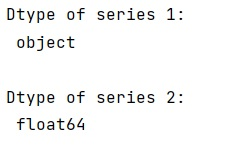# What is the difference between NaN and None?

Learn about the difference between NaN and None in Python.
Submitted by Pranit Sharma, on December 30, 2022

NumPy is an abbreviated form of Numerical Python. It is used for different types of scientific operations in python. Numpy is a vast library in python which is used for almost every kind of scientific or mathematical operation. It is itself an array which is a collection of various methods and functions for processing the arrays.

While creating a DataFrame or importing a CSV file, there could be some NaN values in the cells. NaN values mean "Not a Number" which generally means that there are some missing values in the cell.

## Difference between NaN and None

There might be confusion between a nan value and a none value. Surely None is more descriptive of an empty cell as it has a null value, whereas nan just says that the value read is not a number.

NaN is used as a placeholder for missing data consistently in pandas, consistency is good. I usually read/translate NaN as "missing". The reason to use NaN (over None) is that it can be stored with NumPy’s float64 dtype, rather than the less efficient object dtype while on the other hand, none forces object type, which basically disables all efficiency in NumPy.

The following example will explain with nan values, a numeric value is converted to float data type:

## Python program to demonstrate the difference between NaN and None

```# Import pandas
import pandas as pd

# Import numpy
import numpy as np

# Creating a series with none
none = pd.Series([1, None], dtype=object)

# Creating a series with nan
nan = pd.Series([1, np.nan])

# Display dtypes of both series
print("Dtype of series 1:\n",none.dtype,"\n")
print("Dtype of series 2:\n",nan.dtype,"\n")
```

Output: